#### Two long straight parallel wires, carrying (adjustable) currents I1 and I2, are kept at a distance d apart. If the force ‘F’ between the two wires is taken as ‘positive’ when the wires repel each other and ‘negative’ when the wires attract each other, the graph showing the dependence of ‘F’, on the product  I1I2,  would be : Option 1)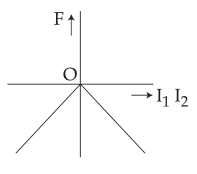Option 2)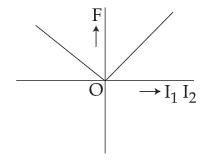Option 3)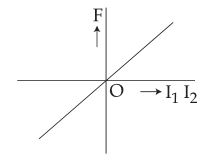Option 4)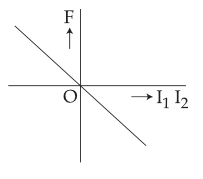As we learnt in

Force between two parallel current carrying conductors -- wherein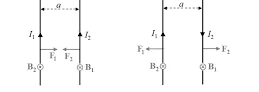I1 and I2 current carrying two parallel wires

a-seperation between two wires

I1 I2 = Positive

Here the force will be attractive and hence it will be taken as negative.

In the second case  I1 I2 = Negative

Here the force will be repulsive and hence it will be taken as positive.

Option 1)Incorrect

Option 2)Incorrect

Option 3)Incorrect

Option 4)Correct

#### Vakul